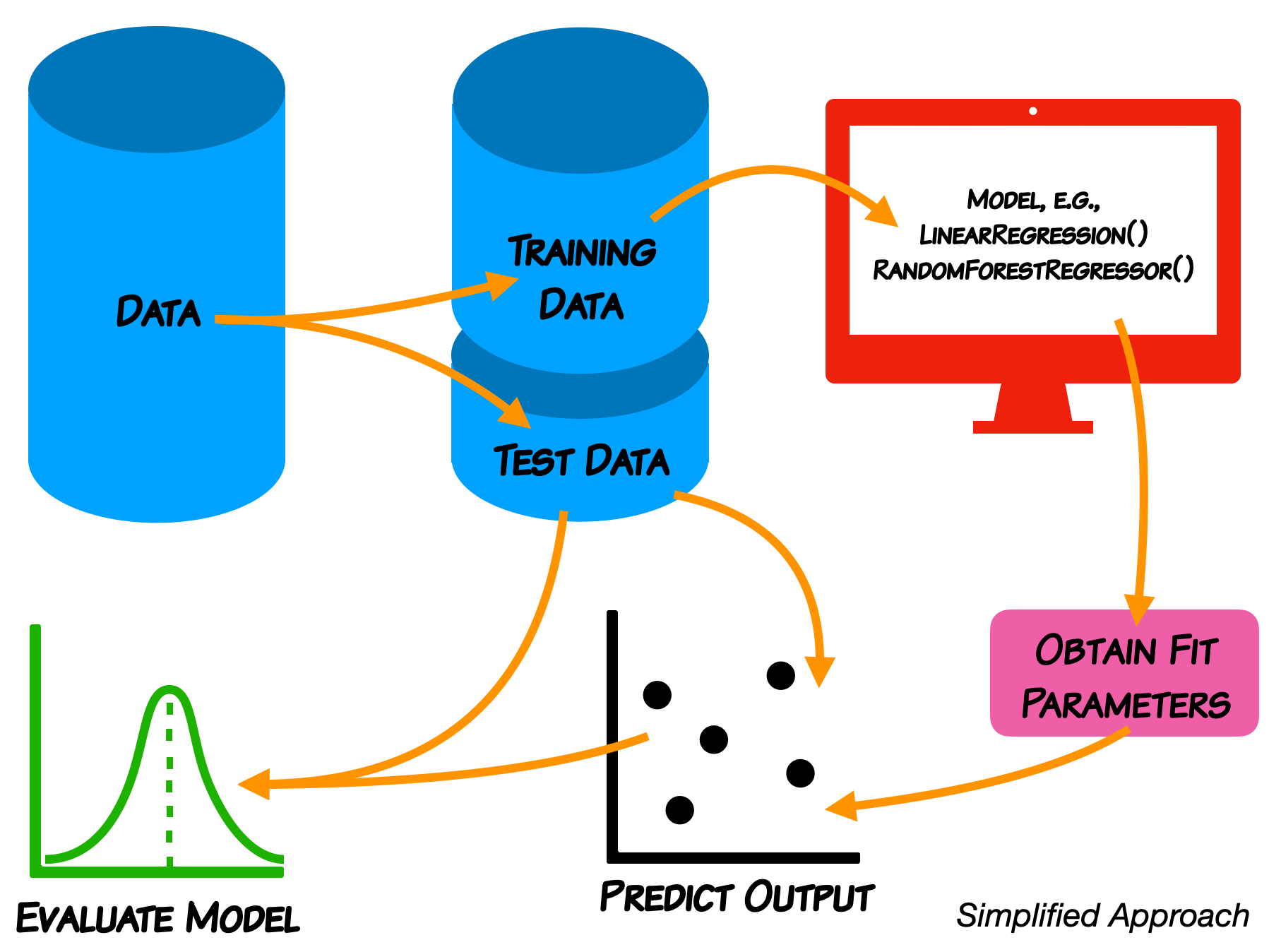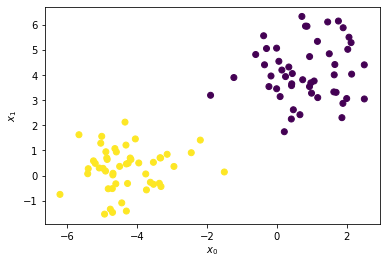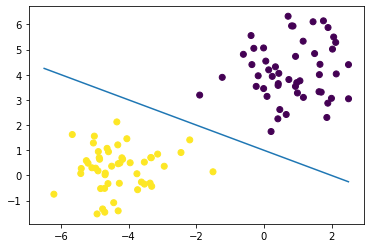# Day 11 - Support Vector Machines¶

## Oct. 13, 2020¶• Midterm will be given Thursday 10/29 in class
• Focus on classification problems (More details on Tuesday; review sheet)
• Read data, clean data, filter data, standardize data, model data, evaluate model with plots
• Open book, note, internet - no chatting with other students
• Changing groups: After the midterm we will put you in new groups for the rest of the semester.
• We will try to keep you with at least one other person from your current group.
• Please complete this MidSemester survey: www.egr.msu.edu/mid-semester-evaluation

# From Pre-Class Assignment¶

## Useful bits¶

• I have a better sense of what an SVM is doing

## Challenging bits¶

• I don't know how much understanding I should try to have about SVM
• I was having trouble making the blobs
• I could not figure out how to get the line of best separation working

# Reminder of the ML Paradigm¶We do not expect you in this class to learn every detail of the models.

# Support Vector Machines¶

• As a classifier, an SVM creates new dimensions from the original data, to be able to seperate the groups along the original features as well as any created dimensions.
• The kernel that we choose tells us what constructed dimensions are available to us.
• We will start with a linear kernel, which tries to construct hyper-planes to seperate the data.
• For 2D, linearly separable data, this is just a line.

We use make_blobs because it gives us control over the data and it's separation; we don't have to clean or standardize it.

## Let's make some blobs¶

In :
##imports
import numpy as np
import matplotlib.pyplot as plt
from sklearn import svm
from sklearn.datasets import make_blobs

X, y = make_blobs(n_samples = 100, n_features=2, centers=2, random_state=3)

## Plot Blobs
plt.scatter(X[:,0], X[:,1], c=y, cmap="viridis")
plt.xlabel(r'$x_0$'); plt.ylabel(r'$x_1$')

Out:
Text(0, 0.5, '$x_1$')## Let's draw a separation line¶

We are just guessing. SVM does this automatically.

In :
## Make guess for separation line
plt.scatter(X[:,0], X[:,1], c=y, cmap="viridis")

xx = np.linspace(-6.5, 2.5)

#yy = -1*xx
#yy = -2 * xx - 1
yy = -0.5 * xx + 1
plt.plot(xx,yy)

Out:
[<matplotlib.lines.Line2D at 0x7ff7f1409750>]